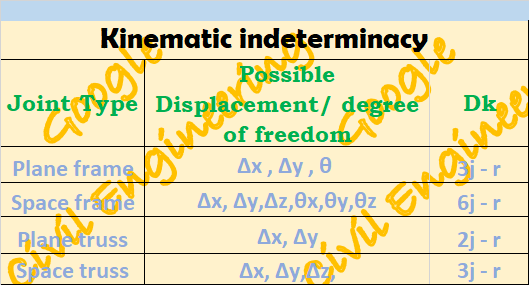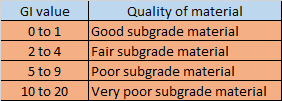## Translate

### How to calculate Kinematic Indeterminacy of a structure

• Kinematic Indeterminacy is defined as the total number of joint displacement present in any structure.
• Kinematic Indeterminacy is also known as unknown degree of freedom.
• Degree of kinematic Indeterminacy is used in case of displacement method of analysis of structure.
• Degree of kinematic Indeterminacy is calculated as the difference between the total possible degree of freedom and known degree of freedom.
• Now,  as support reactions are generated due to restraint of deformation provided by the support.  So,  the number of available support reactions will be equal to the number of restraint joint displacement.
Dk = (total possible degree of freedom) - (Number of available support reaction)Kinematic Indeterminacy, Dk

• Now, in case of internal hinge
• Each internal hinge will add 4 degree of freedom in plane frame case and 9 degree of freedom in case of space frame
• Each joint hinge will add (m'-1) degree of freedom in plane frame case and [3(m'-1)] degree of freedom in case of space frame. Here m' is the the number of members meeting at the hinge joint.
• In case of inextensibility of members, the extension of members are restricted. In this case m number of degree of freedom will be subtracted from the degree of kinematic indeterminacy.

1.This comment has been removed by a blog administrator.

2.This comment has been removed by a blog administrator.

### Group index of soil

Group index of soil is a number used to refer the quality of soil used as a subgrade material in highway construction.  Group index of soil ...